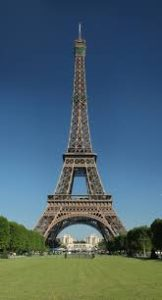Chapter 4: Inequalities

# 4.7 Mathematics in Life: The Eiffel Tower

The Eiffel Tower was completed in 1889 by Gustave Eiffel as the entrance for the world’s fair held in Paris the same year. It has since been visited by an estimated quarter billion people and remains the tallest structure in Paris with a height of 324 m.

Some of the particulars to this construction are that it is comprised of 18,038 pieces of metal held together by some 2.5 million rivets. There are 1,710 steps to the top of the tower, and the iron from which it was fashioned has a mass of 7300 tonnes and requires painting (60 tonnes) every seven years to prevent rusting. The top of the tower (the sun-facing side) can shift up to 18 cm due to thermal expansion. The density of the wrought iron used to create this tower is 7.70 g/cm3 and was allegedly sourced from Reșița in Romania.

Question: If one were to dismantle the Eiffel Tower and melt it to create an iron sphere, what would be its radius?$\text{First, the volume:}$

$\begin{array}{ccrcl} V&=&7,300,000\text{ kg}&\times &\dfrac{1 \text{ cm}^3}{7.70\times 10^{-3}\text{ kg}} \\ \\ V&=&9.48&\times&10^8 \text{ cm}^3 \end{array}$

$\text{Next, the radius:}$

$\begin{array}{rrrrrrl} V&=&\dfrac{4}{3}\pi r^3&\text{or}&r&=&\sqrt{\dfrac{3V}{4\pi}} \\ \\ &&&&&=&\sqrt{\dfrac{3(9.48\times 10^8 \text{ cm}^3)}{4\pi}} \\ \\ &&&&&=&609 \text{ cm} \\ \\ &&&&&=&\sim 6.1 \text{ m} \end{array}$